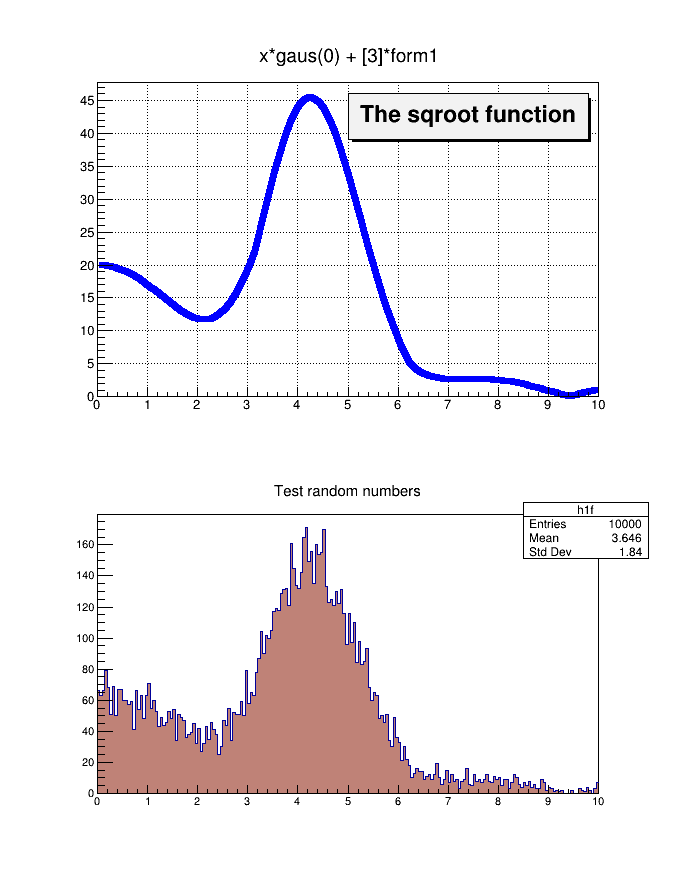# Fillrandom¶

Fill a 1-D histogram from a parametric function.

Author: Rene Brun
This notebook tutorial was automatically generated with ROOTBOOK-izer from the macro found in the ROOT repository on Thursday, May 19, 2022 at 08:10 AM.

Arguments are defined.

In :
int plot=1;

In :
gBenchmark->Start("fillrandom");


A function (any dimension) or a formula may reference an already defined formula

In :
auto form1 = new TFormula("form1","abs(sin(x)/x)");
auto sqroot = new TF1("sqroot","x*gaus(0) + *form1",0,10);
sqroot->SetLineColor(4);
sqroot->SetLineWidth(6);
sqroot->SetParameters(10,4,1,20);


Create a one dimensional histogram (one float per bin) and fill it following the distribution in function sqroot.

In :
auto h1f = new TH1F("h1f","Test random numbers",200,0,10);
h1f->FillRandom("sqroot",10000);


Open a root file and save the formula, function and histogram

In :
TFile myfile("fillrandom.root","RECREATE");
form1->Write();
sqroot->Write();
h1f->Write();


Produce a plot if requested

In :
if (plot) {
TCanvas *c1 = new TCanvas("c1","The FillRandom example",200,10,700,900);

sqroot->Draw();
auto lfunction = new TPaveLabel(5,39,9.8,46,"The sqroot function");
lfunction->Draw();
c1->Update();

h1f->SetFillColor(45);
h1f->Draw();
c1->Update();
}

gBenchmark->Show("fillrandom");

fillrandom: Real Time =   1.05 seconds Cpu Time =   0.41 seconds


Draw all canvases

In :
gROOT->GetListOfCanvases()->Draw()